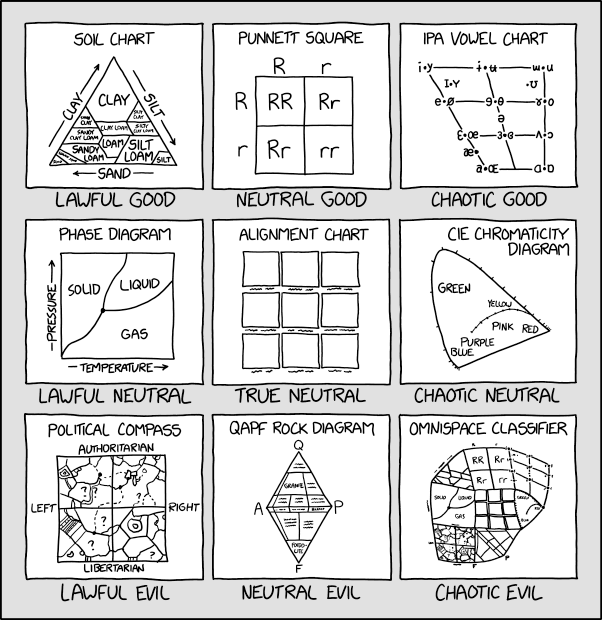← Back

January 6, 2020

# #2251: Alignment Chart Alignment Chart[A 3×3 grid of squares. Each square contains a label at the top and a drawing of a chart, and each square has a caption below it. From left to right, a row at a time:]

Soil Chart

[describe this chart here]

• Clay
• Silty Clay
• […]

Lawful Good

Punnett Square

[describe this chart here]

Neutral Good

IPA Vowel Chart

[describe this chart here]

Chaotic Good

Phase Diagram

[describe this chart here]

Lawful Neutral

Alignment Chart

[A 3×3 grid of nine empty squares, each with an unreadable label below it.]

True Neutral

CIE Chromaticity Diagram

[describe this chart here]

• Green
• Yellow

Chaotic Neutral

Political Compass

[describe this chart here]

Lawful Evil

QAPF Rock Diagram

[The diagram is a rhombus with each corner labeled: ‘Q’ at the top, ‘A’ at the left, ‘P’ at the right, and ‘F’ at the bottom. The diagram is divided into trapezoids and triangles, each with labels. The writing in most subdivisions are unreadable. The readable subdivisions:]

• Granite (around the top left)
• Basalt (just below the right corner)
• Foidolite (at the bottom)

Neutral Evil

Omnispace Classifier

Chaotic Evil

[describe this chart here]